# Path-connected implies connected

Jump to: navigation, search
This article gives the statement and possibly, proof, of an implication relation between two topological space properties. That is, it states that every topological space satisfying the first topological space property (i.e., path-connected space) must also satisfy the second topological space property (i.e., connected space)
View all topological space property implications | View all topological space property non-implications
Get more facts about path-connected space|Get more facts about connected space

## Statement

If a topological space is a path-connected space, it is also a connected space.

## Definitions used

### Connected space

Further information: connected space

A topological space is termed connected if it cannot be expressed as a disjoint union of two nonempty open subsets.

### Path-connected space

Further information: path-connected space

A topological space$X$ is termed path-connected if, for any two points$x,y \in X$, there exists a continuous map$f$ from the unit interval$[0,1]$ to$X$ such that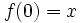$f(0) = x$ and$f(1) = y$.

## Related facts

### Converse

The converse is not true, i.e., connected not implies path-connected.

However, it is true that connected and locally path-connected implies path-connected.

## Proof

### Key ingredient

The key fact used in the proof is the fact that the interval$[0,1]$ is connected. The proof combines this with the idea of pulling back the partition from the given topological space to$[0,1]$.

### Proof details

Given: A path-connected topological space$X$.

To prove:$X$ is connected.

Proof: We do this proof by contradiction. Suppose$X$ is not connected. Then, there exist nonempty disjoint open subsets$U,V \subseteq X$ such that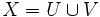$X = U \cup V$. Pick a point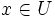$x \in U$ and a point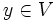$y \in V$.

By assumption, there exists a continuous function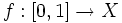$f:[0,1] \to X$ such that$f(0) = x$ and$f(1) = y$. Consider the subsets$f^{-1}(U)$ and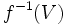$f^{-1}(V)$. These are disjoint in$[0,1]$ and their union is$[0,1]$. By the continuity of$f$, they are both open in$[0,1]$. Finally, since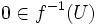$0 \in f^{-1}(U)$ and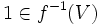$1 \in f^{-1}(V)$, they are both open. We have thus expressed$[0,1]$ as a union of two disjoint nonempty open subsets, a contradiction to the fact that$[0,1]$ is connected. This completes the proof.